# 短視訊中解決音視訊混音出現雜音的問題

### 1 你用過音視訊合成嗎？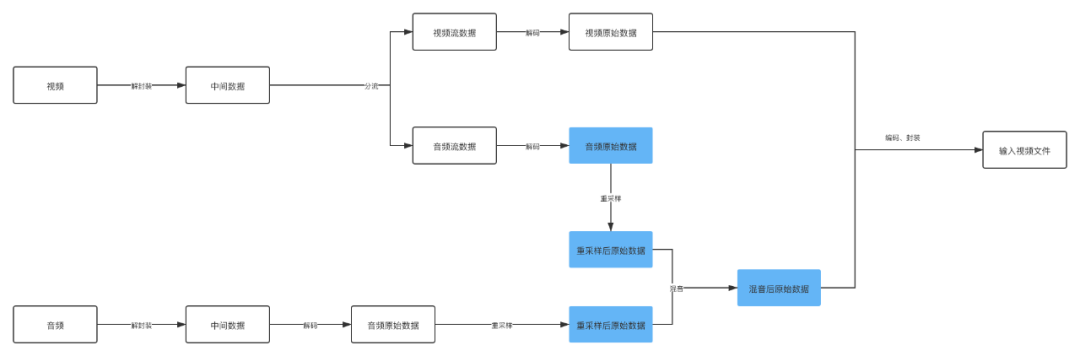### 2 音訊採集的指標

#### 2.1 取樣率

 取樣率 使用場景 8000 Hz 家用電話的取樣率 44100 Hz 音樂CD的取樣率 48000 Hz 標準的音訊取樣率，目前手機大多數採用這個取樣率 96000 Hz 藍光視訊的取樣率

https://en.wikipedia.org/wiki/Sampling_%28signal_processing%29

#### 2.2 取樣位數

• 1位元組：8bit，只能記錄256個數，振幅只能劃分為256個等級。

• 2位元組：16bit，可以細化到65536個數，正常的CD就是這個標準。

• 4位元組：32bit，可以細分到4294967296個數，劃分比較細緻，人耳識別不了這麼細緻的聲音。

#### 2.3 取樣通道數

• mono：單聲道

• stereo：雙聲道

• 4.1聲道：有4個發聲點，前左、前右、後左、後右，同時增加一個低音道，增強對低頻訊號的處理。

• 5.1聲道：基於4.1聲道，同時增加一箇中置單元，這個中置單元負責傳送低於80Hz的聲音訊號，增加了人聲，一般用在電影院裡面。

• 7.1聲道：在聽者的周圍建立起一套前後場相對平衡的聲場，7.1聲道在5.1聲道的基礎上加上了雙路中後置，可以在聽者在任意角度都能聽到一致的聲音。

### 3 聲音的三個基本屬性

#### 3.1 音調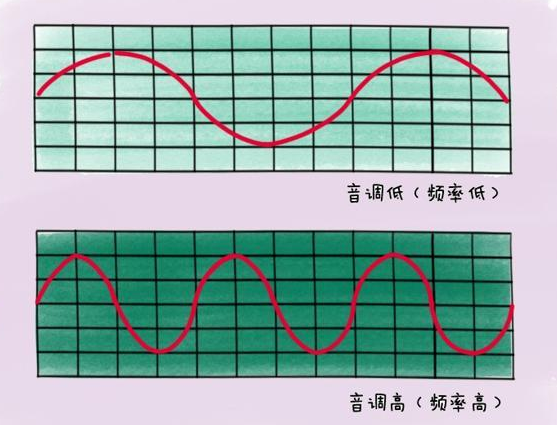#### 3.2 響度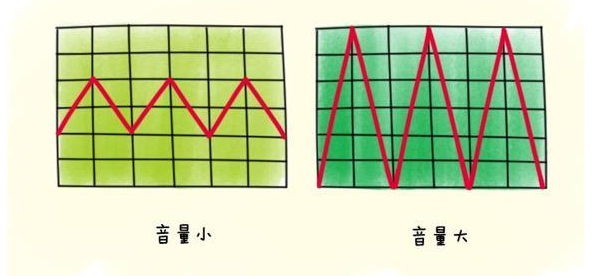#### 3.3 音色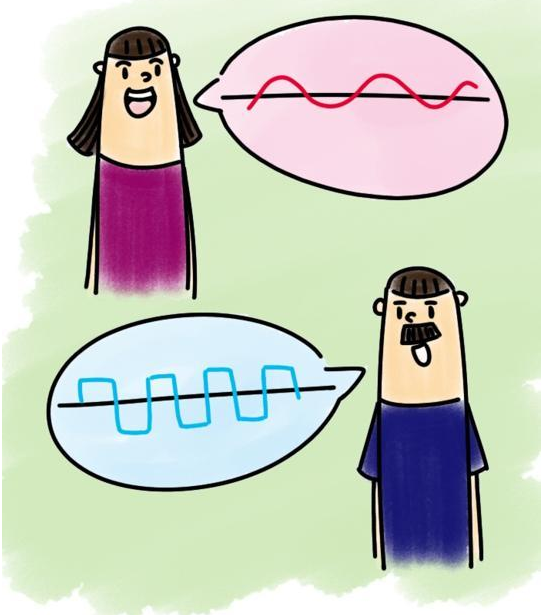### 5 一個雜音的例子

https://github.com/JeffMony/JianYing/blob/main/jeffmony_voice.mp4

``  Duration: 00:00:11.35, start: 0.000000, bitrate: 21123 kb/s``  Stream #0:0(eng): Video: h264 (High) (avc1 / 0x31637661), yuvj420p(pc, bt470bg/bt470bg/smpte170m), 1920x1080, 20341 kb/s, SAR 1:1 DAR 16:9, 30.02 fps, 30 tbr, 90k tbn, 180k tbc (default)``    Metadata:``      rotate          : 90``      creation_time   : 2021-08-04T07:59:58.000000Z``      handler_name    : VideoHandle``      vendor_id       : ``    Side data:``      displaymatrix: rotation of -90.00 degrees``  Stream #0:1(eng): Audio: aac (LC) (mp4a / 0x6134706D), 48000 Hz, stereo, fltp, 320 kb/s (default)``    Metadata:``      creation_time   : 2021-08-04T07:59:58.000000Z``      handler_name    : SoundHandle``      vendor_id       : ``

https://github.com/JeffMony/JianYing/blob/main/output.aac

``  Duration: 00:02:38.18, bitrate: 33 kb/s``  Stream #0:0: Audio: aac (HE-AACv2), 44100 Hz, stereo, fltp, 33 kb/s``

https://github.com/JeffMony/JianYing/blob/main/output1.mp4

``  Duration: 00:00:09.99, start: 0.000000, bitrate: 5938 kb/s``  Stream #0:0(und): Video: h264 (Constrained Baseline) (avc1 / 0x31637661), yuv420p, 720x1280, 5814 kb/s, 30.12 fps, 30 tbr, 10k tbn, 60 tbc (default)``    Metadata:``      handler_name    : VideoHandler``      vendor_id       : ``  Stream #0:1(und): Audio: aac (LC) (mp4a / 0x6134706D), 44100 Hz, mono, fltp, 128 kb/s (default)``    Metadata:``      handler_name    : SoundHandler``      vendor_id       : ``

### 6 問題剖析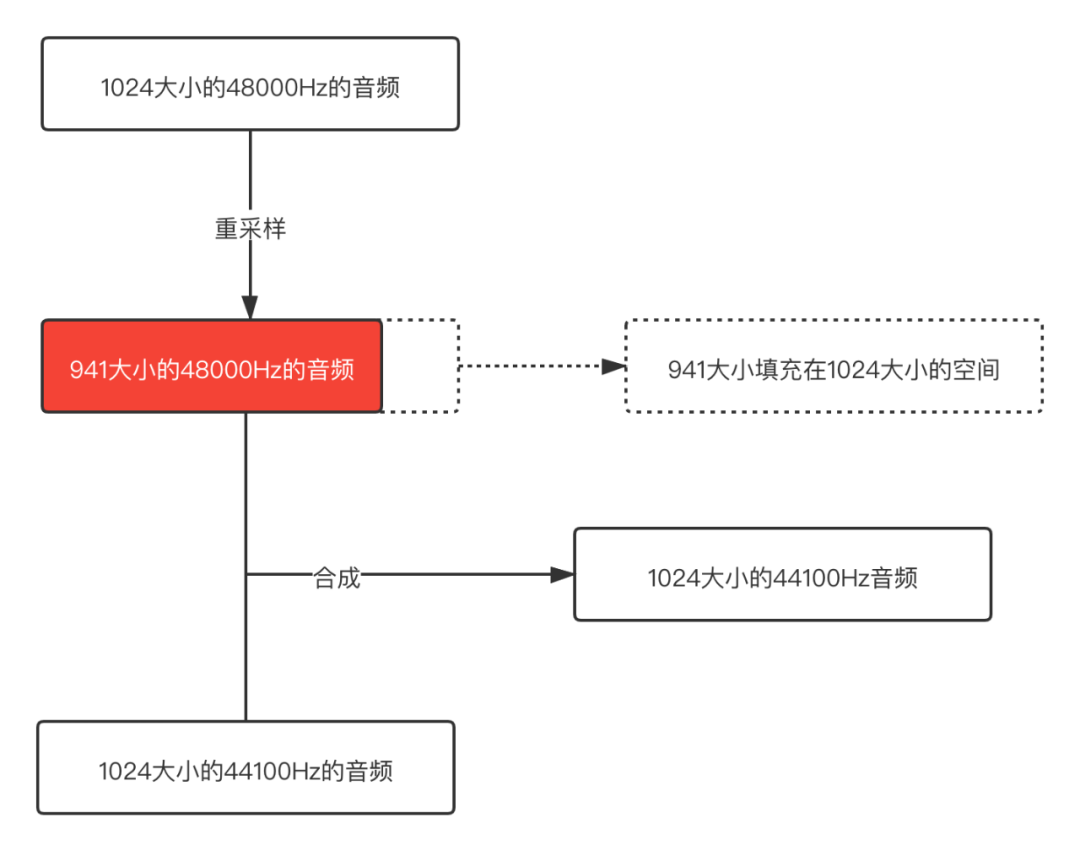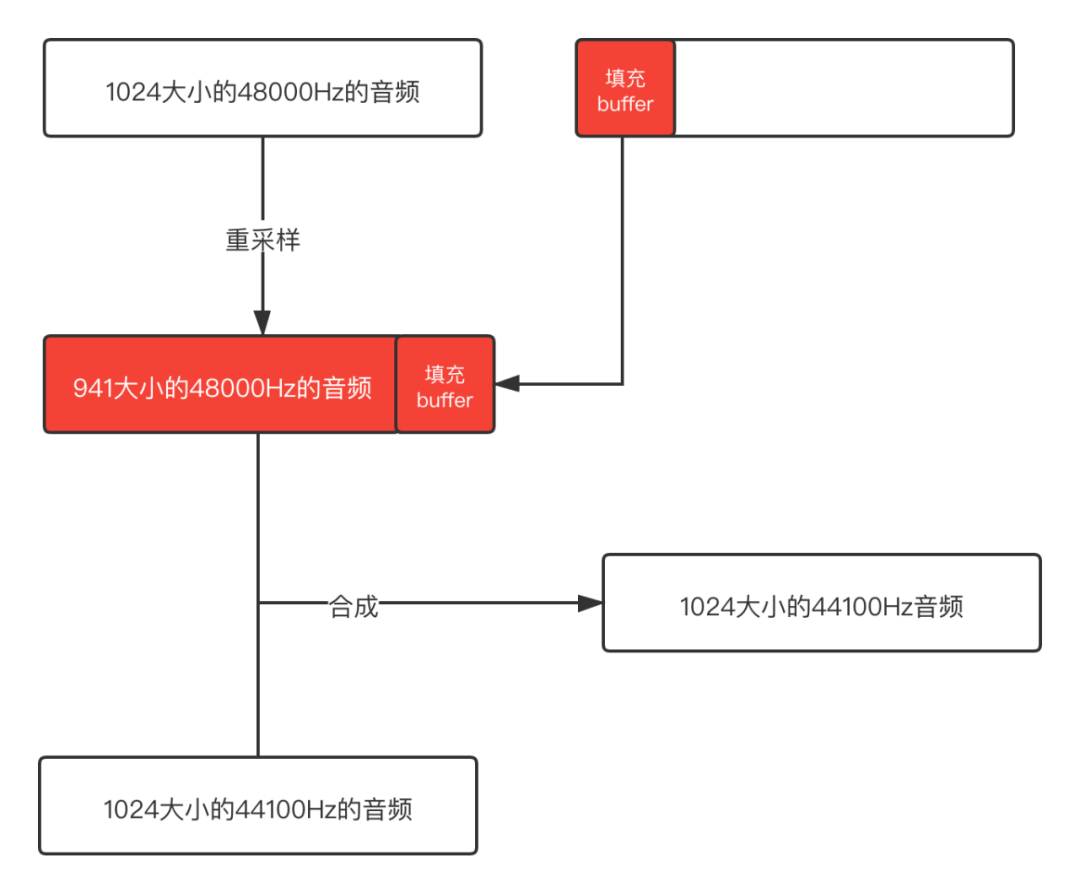https://github.com/JeffMony/JianYing/blob/main/output3.mp4

### 7 混音演算法介紹

#### 7.1 平均法

``public static short[] mixRawAudioBytes(short[][] inputAudios) {``        int coloum = finalLength;//最終合成的音訊長度``        // 音軌疊加``        short[] realMixAudio = new short[coloum];``        int mixVal;``        for (int trackOffset = 0; trackOffset < coloum; ++trackOffset) {``            mixVal = (inputAudios[trackOffset]+inputAudios[trackOffset])/2;``            realMixAudio[trackOffset] = (short) (mixVal);``        }``        return realMixAudio;``    }``

#### 7.2 歸一化

``public static short[] mixRawAudioBytes(short[][] inputAudios) {``        int coloum = finalLength;//最終合成的音訊長度``        float f = divisor;//歸一化因子``        // 音軌疊加``        short[] realMixAudio = new short[coloum];``        float mixVal;``        for (int trackOffset = 0; trackOffset < coloum; ++trackOffset) {``            mixVal = (inputAudios[trackOffset]+inputAudios[trackOffset])*f;``            realMixAudio[trackOffset] = (short) (mixVal);``        }``        return realMixAudio;``    }``

#### 7.3 改進後的歸一化

``    public static short[] mixRawAudioBytes(short[][] inputAudios) {``        int coloum = finalLength;//最終合成的音訊長度``        float f = 1;//衰減因子 初始值為1``        //混音溢位邊界``        int MAX = 32767;``        int MIN = -32768;``        // 音軌疊加``        short[] realMixAudio = new short[coloum];``        float mixVal;``        for (int trackOffset = 0; trackOffset < coloum; ++trackOffset) {``            mixVal = (inputAudios[trackOffset]+inputAudios[trackOffset])*f;``            if (mixVal>MAX){``                f = MAX/mixVal;``                mixVal = MAX;``            }``            if (mixVal<MIN){``                f = MIN/mixVal;``                mixVal = MIN;``            }``            if (f < 1)``            {``                //SETPSIZE為f的變化步長，通常的取值為(1-f)/VALUE,此處取SETPSIZE 為 32   VALUE值可以取 8, 16, 32,64,128.``                f += (1 - f) / 32;``            }``            realMixAudio[trackOffset] = (short) (mixVal);``        }``        return realMixAudio;``    }``

#### 7.4 newlc演算法

if A < 0 && B < 0
Y = A + B - (A * B / (-(2 pow(n-1) -1)))
else
Y = A + B - (A * B / (2 pow(n-1))

``void Mix(char sourseFile[SIZE_AUDIO_FRAME],int number,char *objectFile)  ``{  ``    //歸一化混音  ``    int const MAX=32767;  ``    int const MIN=-32768;  `` ``    double f=1;  ``    int output;  ``    int i = 0,j = 0;  ``    for (i=0;i<SIZE_AUDIO_FRAME/2;i++)  ``    {  ``        int temp=0;  ``        for (j=0;j<number;j++)  ``        {  ``            temp+=*(short*)(sourseFile[j]+i*2);  ``        }                  ``        output=(int)(temp*f);  ``        if (output>MAX)  ``        {  ``            f=(double)MAX/(double)(output);  ``            output=MAX;  ``        }  ``        if (output<MIN)  ``        {  ``            f=(double)MIN/(double)(output);  ``            output=MIN;  ``        }  ``        if (f<1)  ``        {  ``            f+=((double)1-f)/(double)32;  ``        }  ``        *(short*)(objectFile+i*2)=(short)output;  ``    }  ``}``

https://www.git2get.com/av/104606126.html

https://blog.csdn.net/dxpqxb/article/details/78329403